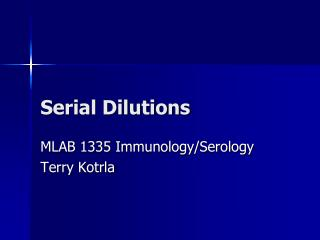DownloadDownload PresentationSerial Dilutions

# Serial Dilutions

Télécharger la présentation## Serial Dilutions

- - - - - - - - - - - - - - - - - - - - - - - - - - - E N D - - - - - - - - - - - - - - - - - - - - - - - - - - -
##### Presentation Transcript

1. Serial Dilutions MLAB 1335 Immunology/Serology Terry Kotrla

2. Introduction • Many of the laboratory procedures involve the use of dilutions. • It is important to understand the concept of dilutions, since they are a handy tool used throughout all areas of the clinical laboratory. • These dilutions have to be considered as they make a quantitative difference in what is going on.

3. Serial Dilutions • A serial dilution is any dilution where the concentration decreases by the same quantity in each successive step. • Serial dilutions are mutiplicative.

4. What Does This Mean?? • If a solution has a 1/10 dilution the number represents 1 part of the patient sample added to 9 parts of diluent. • So the volumes used would be 10-1= 9. • This represents 1 part patient sample added to 9 parts of diluent.

5. Dilutions • If a 1/8 dilution of the stock solution is made followed by a 1/6 dilution what is the final dilution. • The final dilution is: 1/8 x 1/6 = 1/48 • These type of dilutions are trickier and not used very frequently in the clinical lab.

6. Doubling Dilutions • “Doubling dilutions” are very popular. • This is a series of ½ dilutions. Each successive tube will ½ the amount of the original concentrated solution. • If this is done 6 times this is what you would end up with:

7. Doubling Dilution 6 Times • 1st dilution = 1 /2 • 2nd dilution = 1 /2 x 1 /2 = 1/4 • 3rd dilution = 1/4 x 1 /2 = 1/8 • 4th dilution = 1/8 x 1 /2 = 1/16 • 5th dilution = 1/16 x 1 /2 - 1/32 • 6th dilution = 1/32 x 1 /2 = 1/64 • This results in a series of dilutions, each a doubling dilution of the previous one

8. Dilution Factor • The dilution factor is the final uses the formula volume/aliquot volume. • EXAMPLE: What is the dilution factor if you add 0.1 mL aliquot of a specimen to 9.9 mL of diluent? • The final volume is equal to the aliquot volume PLUS the diluent volume: 0.1 mL + 9.9 mL = 10 mL • The dilution factor is equal to the final volume divided by the aliquot volume: 10 mL/0.1 mL = 1:100 dilution

9. Practice • Problem: What is the dilution factor when 0.2 mL is added to 3.8 mL diluent?

10. Set Up The Problem • dilution factor = final volume/aliquot volume • 0.2 +3.8 = 4.0 total volume • 4.0/0.2 = 1:20 dilution

11. Problem Continued • Remember that serial dilutions are always made by taking a set quantity of the initial dilution and adding it successively to tubes with the same volume. • So each successive dilution would be multiplied by the dilution factor.

12. Problem Continued • So in the above problem all successive tubes would have 3.8 mLs of diluent. • You would then transfer 0.2 of the initial diluted sample into the next tube, mix transfer 0.2, mix and so on. • If you had 4 tubes what would be the final dilution of tube 4?

13. Solving the Problem - *Calculate DF of tube 1

14. Solving the Problem • Or if you simply wanted to know the dilution of the final tube you could just multiply them together: • 1/20 x 1/20 x 1/20 x 1/20 = 1:160,000

15. Next • What if we added the indicator system to the test system? • You must create additional rows, if you are showing your work, to take into consideration the dilutional effect that will have on the dilution. • When an indicator is added it is CRITICAL to remove an aliquot from the last tube.